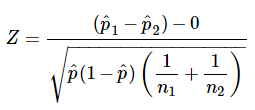# SiteGainer Stats Engine

Classical statistical techniques have been the foundation of many important areas of inquiry including clinical drug trials, manufacturing design, and most areas of scientific inquiry ranging from psychology to particle physics for hundreds of years. As such they are appropriate and satisfactory for decision making in conversion rate optimization in digital marketing.

The proper application of frequentist statistics to optimization decisions yields solid, highly interpretable results that conversion rate optimization professionals can use to make everyday business decisions.

SiteGainer’s stats engine requires users to calculate in advance the sample size for each test with the assumption that each test is a superiority test better known as a one-tailed test. Since 95% of statistical hypothesis in conversion rate optimization are based on research hypotheses that are seeking to determine if a variation is better than the original this is the most appropriate application of frequentist statistical methods for conversion rate optimization.

A link to our sample size calculator

The SiteGainer stats engine uses the Z-test for the difference between two proportions. The underlying assumptions necessary for the application of this test are the following:

Samples are independent of one another
The sample size is >30
Data is normally distributed
Data is randomly selected from the populations with each data point having an equal probability of being selected.
Sample sizes should be roughly equal

The formula for computing the test statistic is:Where

p = (P1 + P2 ) / (N1 + N2)

P1 and P2 are the proportions of the original and the variation and N1 and N2 are the sample sizes of the original and variation respectively. P is

The SiteGainer stats engine will use the Z score to calculate the significance which is compared to the user’s required significance prior to the test start and based on the user’s individual business requirements.